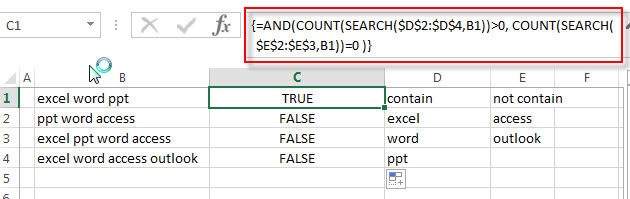# Check if Cell Contains Certain Values but do not Contain Others Values

In the previous post, we only talked that how to check a cell if contains one of several values from a range in excel. And this post explains that how to check a cell if it contains certain values or contains one of several values but do not contain other certain values in another range or a list in excel.

## Check if Cell Contains Certain Values but do not Contain Others Values

Supposing that you have a list of text strings in the range B1:B3 and you need to check each Cell that if it contains one of several values in a range D2:D4 but do not contains any of values in range E2:E3.  If TRUE, then returns TRUE value, otherwise, returns FALSE value.

To check a cell to see if it contains certain string but do not contain others. You can use a combination of the AND function, the COUNT function and the SEARCH function to create a new array formula as follows:

`=AND(COUNT(SEARCH(\$D\$2:\$D\$4,B1))>0, COUNT(SEARCH(\$E\$2:\$E\$3,B1))=0 )`

Let’s see how this formula works:

= SEARCH(\$D\$2:\$D\$4,B1)

The SEACH function returns position of the first character of find_text in a text string. And this formula will search each value in the range D2:D4 inside within_text in Cell B1, then returns position of each text string in Cell B1, so it will return an array result like this:

`{1;7;12}`

= SEARCH(\$E\$2:\$E\$3,B1)

This SEARCH formula will search each value in range E2:E3 inside within_text in Cell B1, and then returns position of the first position of each find_text in Cell B1. When no match is found, the SEARCH function will return the #VALUE error. So it will return an array result like this:

`{#VALUE!;#VALUE!;1}`

=COUNT(SEARCH(\$D\$2:\$D\$4,B1))>0

The COUNT function will count the number of cells that contain numbers in an array returned by the SEARCH function. If it is greater than 0, then it indicated that at least one values is found in Cell B1.

=COUNT(SEARCH(\$E\$2:\$E\$4,B1))=0

If the number of cells that contain numbers is equal to 0, then it means that none of values can be found in Cell B1.

=AND(COUNT(SEARCH(\$D\$2:\$D\$4,B1))>0, COUNT(SEARCH(\$E\$2:\$E\$4,B1))=0 )

If both two COUNT function return TRUE value, the AND function returns TRUE. And if either COUNT function is FALSE, this formula returns FALSE.### Related Functions

• Excel SEARCH function
The Excel SEARCH function returns the number of the starting location of a substring in a text string.The syntax of the SEARCH function is as below:= SEARCH  (find_text, within_text,[start_num])…
• Excel COUNT function
The Excel COUNT function counts the number of cells that contain numbers, and counts numbers within the list of arguments. It returns a numeric value that indicate the number of cells that contain numbers in a range…
• Excel AND function
The Excel AND function returns TRUE if all of arguments are TRUE, and it returns FALSE if any of arguments are FALSE.The syntax of the AND function is as below:= AND (condition1,[condition2],…)…